Question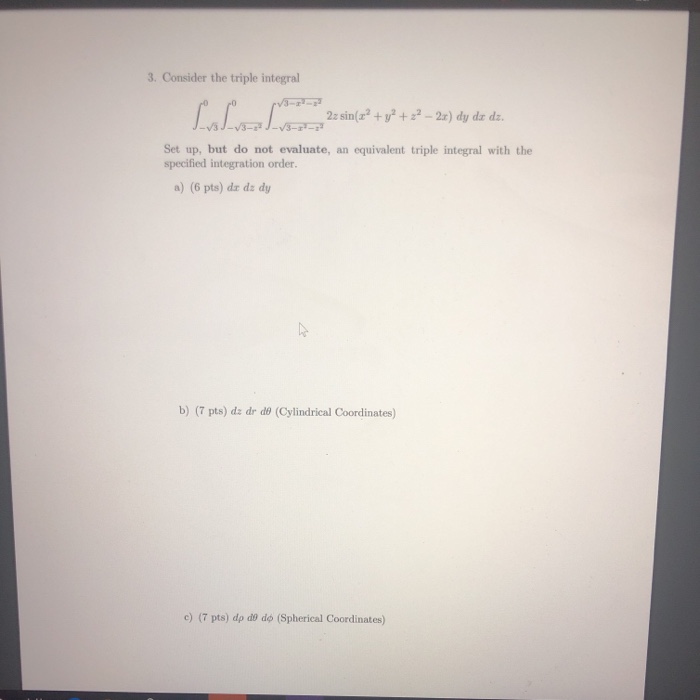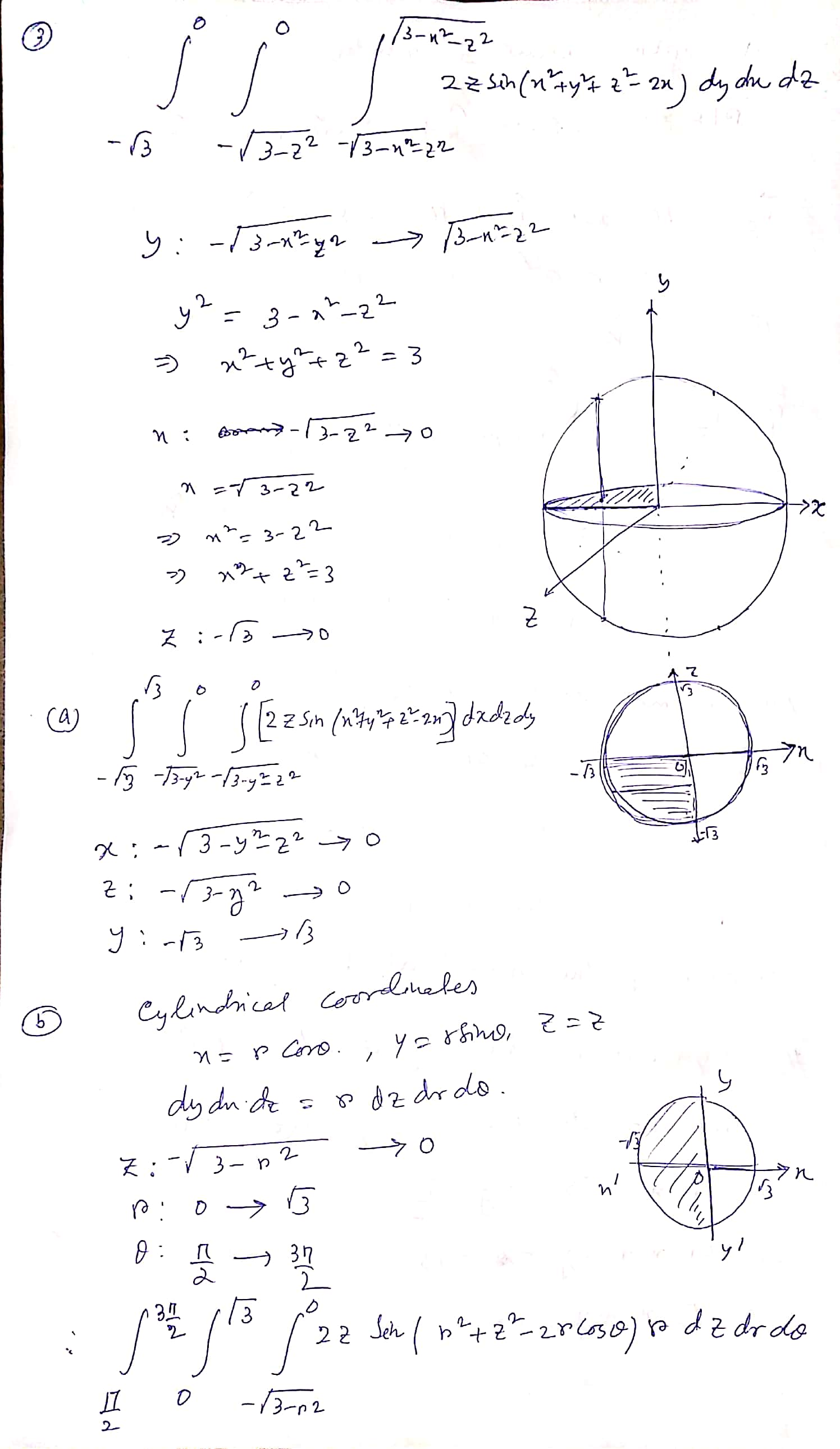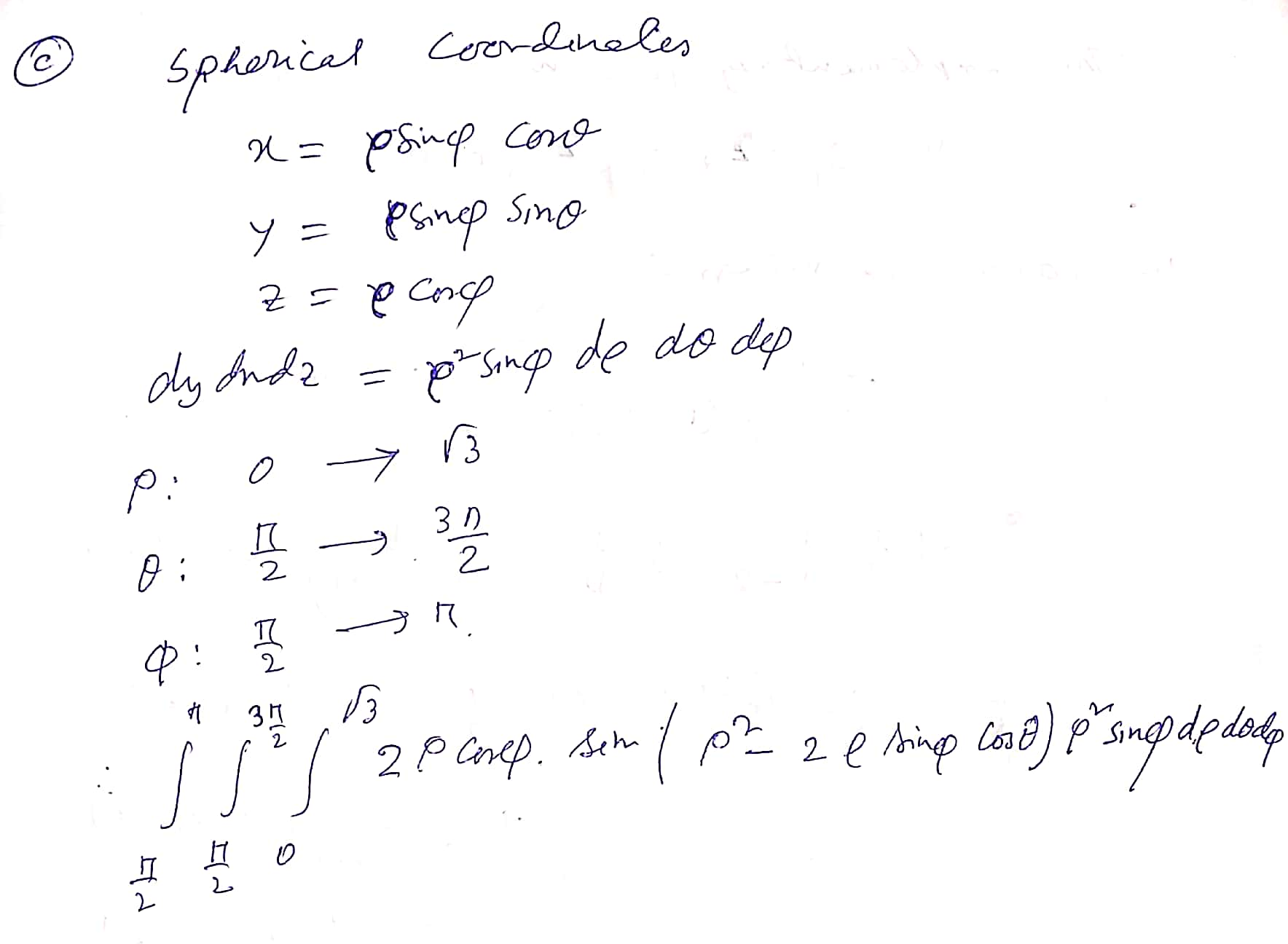#### Earn Coins

Coins can be redeemed for fabulous gifts.

Similar Homework Help Questions
• ### Consider the triple integral LLL 3- 2z sin(x² + y2 + 22 - 2x) dy do...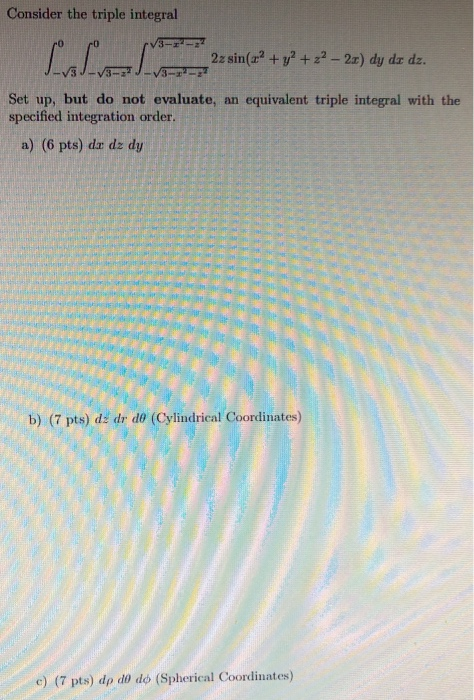Consider the triple integral LLL 3- 2z sin(x² + y2 + 22 - 2x) dy do dz. -3-2- Set up, but do not evaluate, an equivalent triple integral with the specified integration order. a) (6 pts) do dz dy b) (7 pts) dz dr do (Cylindrical Coordinates) c) (7 pts) dp do do (Spherical Coordinates)

• ### 16. o integrad [**** The triple da dy dz describes the solid pictured at right. Rewrite...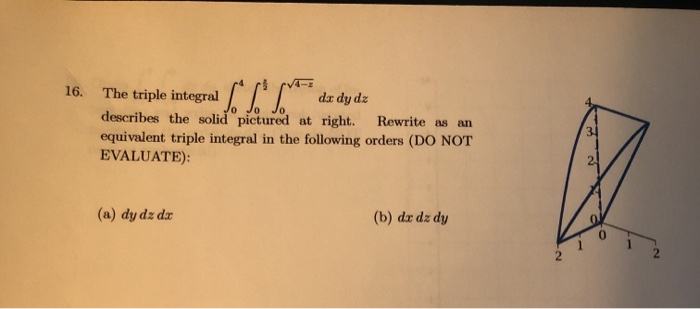16. o integrad [**** The triple da dy dz describes the solid pictured at right. Rewrite as an equivalent triple integral in the following orders (DO NOT EVALUATE): 31 (a) dy dz dx (b) du dz dy 2. 16-2 21. Given dy da, 16- (a) Sketch the region of integration and write an equivalent iterated integral in the order dx dy. (You do not need to evaluate it!) (b) Now write it as an equivalent iterated integral in polar coordinates....

• ### 6. (4 pts) Consider the double integral∫R(x2+y)dA=∫10∫y−y(x2+y)dxdy+∫√21∫√2−y2−√2−y2(x2+y)dxdy.(a) Sketch the region of integration R in Figure 3.(b)...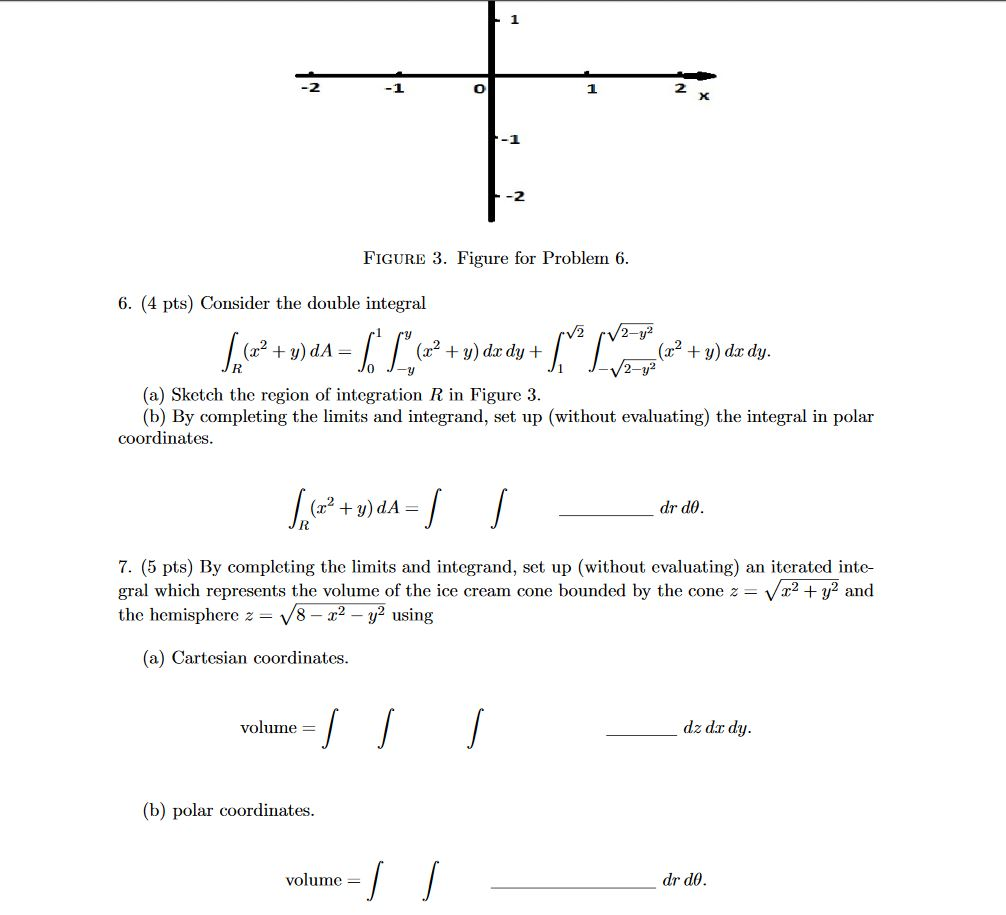6. (4 pts) Consider the double integral∫R(x2+y)dA=∫10∫y−y(x2+y)dxdy+∫√21∫√2−y2−√2−y2(x2+y)dxdy.(a) Sketch the region of integration R in Figure 3.(b) By completing the limits and integrand, set up (without evaluating) the integral in polar coordinates.∫R(x2+y)dA=∫∫drdθ.7. (5 pts) By completing the limits and integrand, set up (without evaluating) an iterated inte-gral which represents the volume of the ice cream cone bounded by the cone z=√x2+y2andthe hemisphere z=√8−x2−y2using(a) Cartesian coordinates.volume =∫∫∫dz dxdy.(b) polar coordinates.volume =∫∫drdθ. -1 -2 FIGURE 3. Figure for Problem 6. 6. (4 pts)...

• ### 6. (4 pts) Consider the double integral∫R(x2+y)dA=∫10∫y−y(x2+y)dxdy+∫√21∫√2−y2−√2−y2(x2+y)dxdy.(a) Sketch the region of integrationRin Figure 3.(b) By completing...6. (4 pts) Consider the double integral∫R(x2+y)dA=∫10∫y−y(x2+y)dxdy+∫√21∫√2−y2−√2−y2(x2+y)dxdy.(a) Sketch the region of integrationRin Figure 3.(b) By completing the limits and integrand, set up (without evaluating) the integral in polar coordinates. -1 -2 FIGURE 3. Figure for Problem 6. 6. (4 pts) Consider the double integral V2 /2-y² + = (x2 + y) dx dy + + y) do dy. 2-y2 (a) Sketch the region of integration R in Figure 3. (b) By completing the limits and integrand, set up (without evaluating)...

• ### 16. Question Details LarCalc11 14.6.017. (3865000) Set up a triple integral for the volume of the...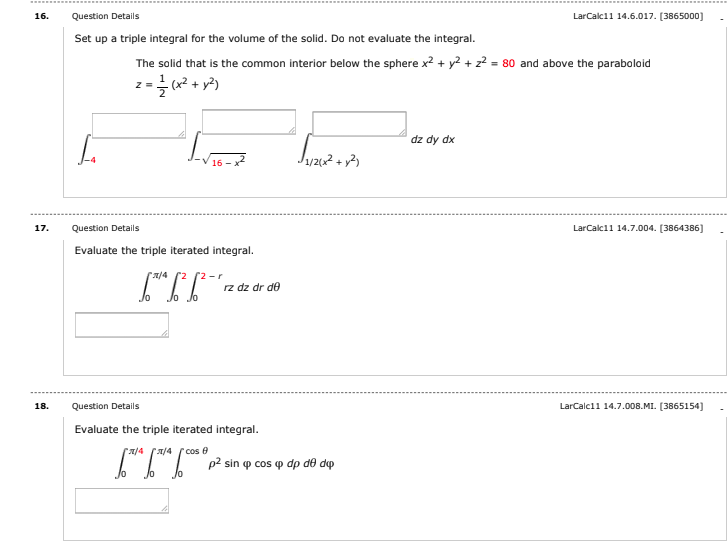16. Question Details LarCalc11 14.6.017. (3865000) Set up a triple integral for the volume of the solid. Do not evaluate the integral. The solid that is the common interior below the sphere x2 + y2 + 2+ = 80 and above the paraboloid z = {(x2 + y2) dz dy dx L J1/2012 + y2 Super 17. LarCalc11 14.7.004. (3864386] Question Details Evaluate the triple iterated integral. 6**6*6*2 2/4 2 2r rz dz dr de Jo lo 18. Question Details...

• ### Consider the solid enclosed by x2 + y2 + z2 = 2z and z2 = 3(x2 + y2) in the 1st octant.

Consider the solid enclosed by x2 + y2 + z2 = 2z and z2 = 3(x2 + y2) in the 1st octant. a) Set up a triple integral using spherical coordinates that can be used to find the volume of the solid. Clearly indicate how you get the limits on each integral used. b) Using technology, or otherwise, evaluate the triple integral to find the volume of the solid.

• ### 6. (4 pts) Consider the double integral∫R(x2+y)dA=∫10∫y−y(x2+y)dxdy+∫√21∫√2−y2−√2−y2(x2+y)dxdy.(a) Sketch the region of integration R in Figure 3.(b)...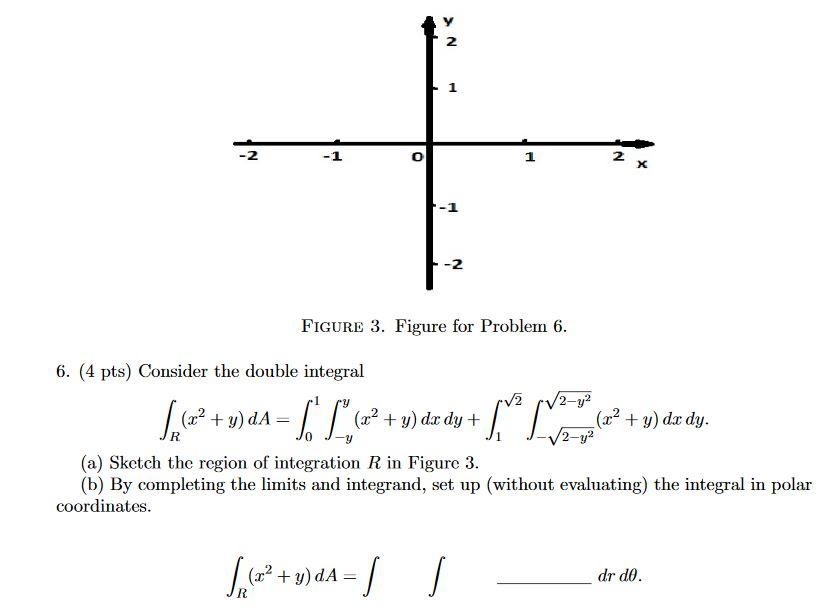6. (4 pts) Consider the double integral∫R(x2+y)dA=∫10∫y−y(x2+y)dxdy+∫√21∫√2−y2−√2−y2(x2+y)dxdy.(a) Sketch the region of integration R in Figure 3.(b) By completing the limits and integrand, set up (without evaluating) the integral in polar coordinates. 2 1 2 X -2 FIGURE 3. Figure for Problem 6. 6. (4 pts) Consider the double integral V2 2-y2 (2? + y) dA= (32 + y) dx dy + (x2 + y) dx dy. 2-y? (a) ketch the region of integration R in Figure 3. (b) By completing...

• ### 4. (20 points) Use integration in spherical coordinates to evaluate the triple integral where E i...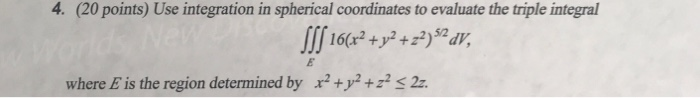4. (20 points) Use integration in spherical coordinates to evaluate the triple integral where E is the region determined by x2 +y2 + z's 2z. 4. (20 points) Use integration in spherical coordinates to evaluate the triple integral where E is the region determined by x2 +y2 + z's 2z.

• ### Covert 1 = 83 84-** S*-**-»* dz dydx to an equivalent integral in cylindrical coordinates. Let...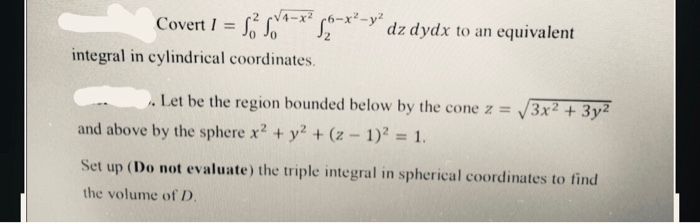Covert 1 = 83 84-** S*-**-»* dz dydx to an equivalent integral in cylindrical coordinates. Let be the region bounded below by the cone z = and above by the sphere x2 + y2 + (z - 1)2 = 1. |3x² + 3y² Set up (Do not evaluate) the triple integral in spherical coordinates to find the volume of D.

• ### Question Use cylindrical coordinates to set up the triple integral needed to find the volume of...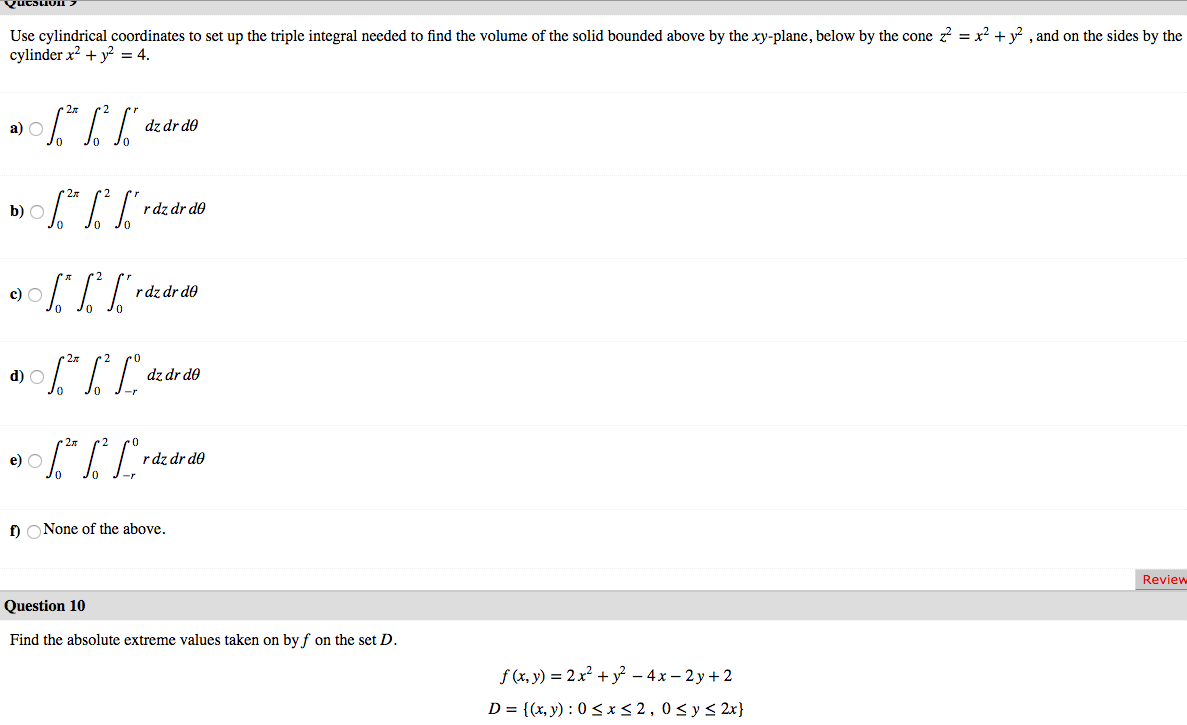Question Use cylindrical coordinates to set up the triple integral needed to find the volume of the solid bounded above by the xy-plane, below by the cone z = x2 + y2 , and on the sides by the cylinder x2 + y2 = 4. a) 06.* %* ["dz dr do b) \$* * S*rde de do JO 0% ] raz dr do a) \$** [Lºdz dr do 0906.*|*Lºrdz dr do 2 po dz dr do Jo J- O J-...